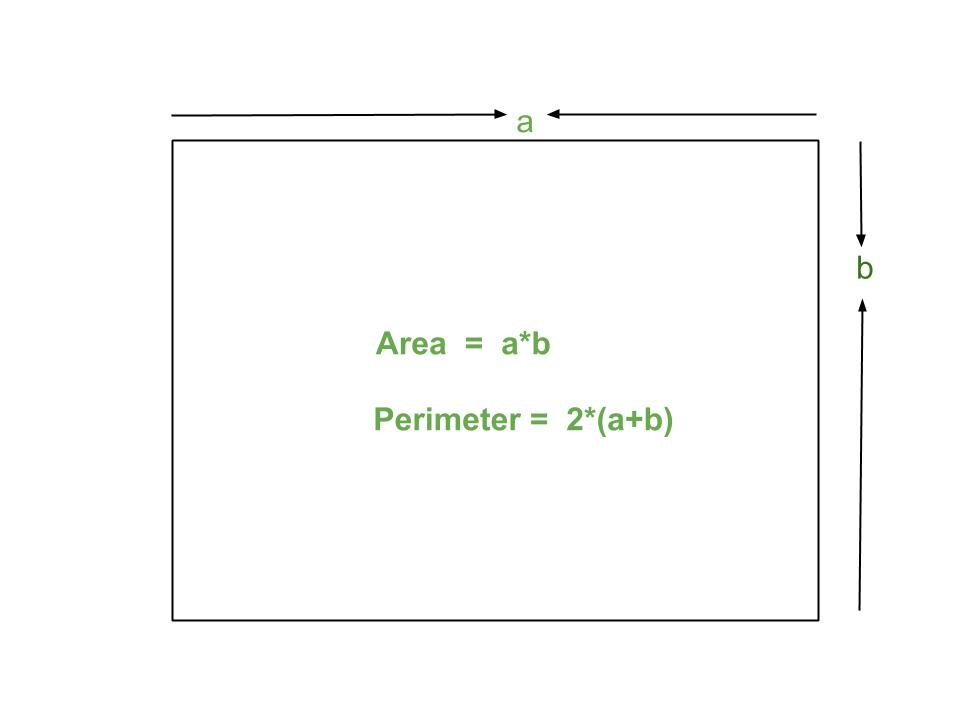Related Articles
Program for Area And Perimeter Of Rectangle
• Difficulty Level : Easy
• Last Updated : 24 Mar, 2021

A rectangle is a flat figure in a plane.It has four sides and four equal angles of 90 degree each.In rectangle all four sides are not of equal length like square, sides opposite to each other have equal length.Both diagonals of rectangle have equal length.Examples:

```Input : 4 5
Output : Area = 20
Perimeter = 18

Input : 2 3
Output : Area = 6
Perimeter = 10```

Formulae :

```Area of rectangle : a*b
Perimeter of ractangle: 2*(a + b)```

## C++

 `// CPP program to find area``// and perimeter of rectangle``#include``using` `namespace` `std;` `// Utility function``int` `areaRectangle(``int` `a, ``int` `b)``{``   ``int` `area = a * b;``   ``return` `area;``}` `int` `perimeterRectangle(``int` `a, ``int` `b)``{``   ``int` `perimeter = 2*(a + b);``   ``return` `perimeter;``}` `// Driver program``int` `main()``{``  ``int` `a = 5;``  ``int` `b = 6;``  ``cout << ``"Area = "` `<< areaRectangle(a, b) << endl;``  ``cout << ``"Perimeter = "` `<< perimeterRectangle(a, b);``  ``return` `0;``}`

## Java

 `// Java program to find area``// and perimeter of rectangle``import` `java.io.*;` `class` `Geometry {` `    ``// Utility function``    ``static` `int` `areaRectangle(``int` `a, ``int` `b)``    ``{``       ``int` `area = a * b;``       ``return` `area;``    ``}` `    ``static` `int` `perimeterRectangle(``int` `a, ``int` `b)``    ``{``       ``int` `perimeter = ``2``*(a + b);``       ``return` `perimeter;``    ``}``    ` `    ``// Driver Function``    ``public` `static` `void` `main (String[] args) {` `        ``int` `a = ``5``;``        ``int` `b = ``6``;``        ``System.out.println(``"Area = "``+ areaRectangle(a, b));``        ``System.out.println(``"Perimeter = "``+ perimeterRectangle(a, b));` `    ``}``}` `// This code is contributed by Chinmoy Lenka`

## Python3

 `# Python3 code to find area``# and perimeter of rectangle` `# Utility function``def` `areaRectangle(a, b):``    ``return` `(a ``*` `b)` `def` `perimeterRectangle(a, b):``    ``return` `(``2` `*` `(a ``+` `b))` `# Driver function``a ``=` `5``;``b ``=` `6``;``print` `(``"Area = "``, areaRectangle(a, b))``print` `(``"Perimeter = "``, perimeterRectangle(a, b))` `# This code is contributed by 'saloni1297'.`

## C#

 `// C# program to find area``// and perimeter of rectangle``using` `System;` `class` `GFG {` `    ``// Utility function``    ``static` `int` `areaRectangle(``int` `a, ``int` `b)``    ``{``        ``int` `area = a * b;``        ``return` `area;``    ``}` `    ``static` `int` `perimeterRectangle(``int` `a, ``int` `b)``    ``{``        ``int` `perimeter = 2 * (a + b);``        ``return` `perimeter;``    ``}` `    ``// Driver Function``    ``public` `static` `void` `Main()``    ``{` `        ``int` `a = 5;``        ``int` `b = 6;``        ` `        ``Console.WriteLine(``"Area = "``                      ``+ areaRectangle(a, b));``        ``Console.WriteLine(``"Perimeter = "``                 ``+ perimeterRectangle(a, b));``    ``}``}` `// This code is contributed by vt_m.`

## PHP

 ``

## Javascript

 ``

Output :

```Area = 30
Perimeter = 22```

This article is contributed by Saloni Gupta. If you like GeeksforGeeks and would like to contribute, you can also write an article using contribute.geeksforgeeks.org or mail your article to contribute@geeksforgeeks.org. See your article appearing on the GeeksforGeeks main page and help other Geeks.
Please write comments if you find anything incorrect, or you want to share more information about the topic discussed above.

Attention reader! Don’t stop learning now. Get hold of all the important DSA concepts with the DSA Self Paced Course at a student-friendly price and become industry ready.

My Personal Notes arrow_drop_up